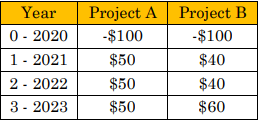top of page
Search

# How to Calculate Net Present Value (NPV)

Updated: Dec 28, 2021

NPV provides an indication to the benefit that is going to be created by the project in question. This formula is leveraged when an organization is determining which projects are worthwhile to invest in. Projects to be worked on should provide value that's more than the cost to implement the project.

With the NPV formula, the final result is derived by: calculating the present value of cash flows, discounting this amount by the hurdle rate, and then subtracting the initial investment (cost) to attain the final result. If the result is positive, then we should proceed with the project. If the result is negative, then we should not move forward.

A word of caution is the NPV’s hurdle rate is only an estimate so there may be uncertainty in NPV and, therefore, your decision to choose this project. Further, large-scale projects normally have NPV’s very different from smaller project’s NPV’s. If you use NPV, the scale of the project must be considered.

## Net Present Value Example

Two projects are being considered – Project A and Project B. Below are the estimated cash flows for each project. Note that Year 0 is the year that the project is undertaken and there are costs realized to execute this project. That’s why there is a negative value here. Subsequent years will have the cash flows as a direct result of implementing the project. This is the income to the organization that the project realizes.We will assume that the required rate of return is 10% which represents the percentage of return expected by executive management.

Now we will decide which project is better to undertake. We’ll first start with Project A.

The formula to discover present value is:

PV (present value) = PMT/(1+i)n

PMT = Payment

i = Expected rate of return

n = Number of years from time of payment (year 0)

The NPV for Project A can be written as:

NPV = \$50/1.1 + \$50/(1.1)2 + \$50/(1.1)3 - \$100 = \$24.34.

In regards to project B, the NPV formula can be written as:

NPV = \$40/1.1 + \$40/(1.1)2 + \$60/(1.1)3 - \$100 = \$14.50

If both of these projects were part of the same program, then both projects can be undertaken as they both provide positive value to the organization. However, if you only could choose 1 project then you’d select project A because it provides more value.

## Use MS Excel to Calculate NPV

There is another way to calculate NPV without needing to use mathematical formulas or leveraging an HP-12C Finance calculator. You can use MS Excel which I will now detail below.

The first thing to do is to set up your Excel sheet as shown below:There is an NPV formula in Excel that is highlighted in cell H5. The first argument in this function is \$A\$1 which represents the expected rate of return. This is an absolute reference because we can list multiple projects underneath and leverage this same percentage for each project without typing the expected rate of return over and over. Another benefit is that, if we change the expected rate of return, we can instantly see the effect on the NPV of the projects we’re evaluating.

The range C5:G5 represents the cash flows ONLY and not the original expense of undertaking the project.

Cell B5 represents the cost of undertaking the project in “Year 0.” Make sure that you enter a negative number in cell B5 as this is an outflow (cost) for the project. Taking a look at the NPV formula, you’ll notice that you’re adding cell B5 instead of subtracting it. In MS Excel we use the add operator since the value you’re entering in cell B5 is negative.